# FM-Indexes and Backwards Search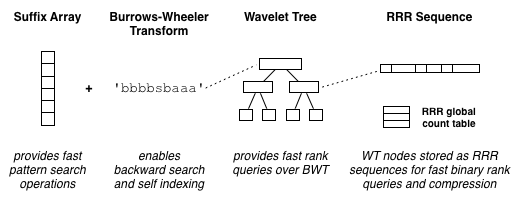Last time (way back in June! I have got to start blogging consistently again) I discussed a gorgeous data structure called the Wavelet Tree. When a Wavelet Tree is stored using RRR sequences, it can answer rank and select operations in $\mathcal{O}(\log{A})$ time, where A is the size of the alphabet. If the size of the alphabet is $2$, we could just use RRR by itself, which answers rank and select in $\mathcal{O}(1)$ time for binary strings. RRR also compresses the binary strings, and hence compresses a Wavelet Tree which is stored using RRR.

So far so good, but I suspect rank and select queries seem to be of limited use right now (although once you are familiar with the available structures, applications show up often). One of the neatest uses of rank that I’ve seen is in substring search, which is certainly a wide reaching problem (for a very recent application to genome assembly, see Jared Simpson’s paper from 2010 called Efficient construction of an assembly string graph using the FM-index).

Note that arrays and strings are one-based (not zero-based).

# Suffix Arrays

There is a variety of Suffix Array construction algorithms, including some $\mathcal{O}(N)$ ones (Puglisi et al. 2007). However, I will explain it from the most common (and intuitive) angle.

In its simplest form, a suffix array can be constructed for a string $S[1..N]$ like so:

1. Construct an array of pointers to all suffixes $S[1..N], S[2..N], …, S[N..N]$.
2. Sort these pointers by the lexicographical (i.e. alphabetical) ordering of their associated suffixes.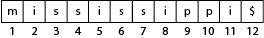For example, the sorting of the string 'mississippi' with terminating character $ would look like this: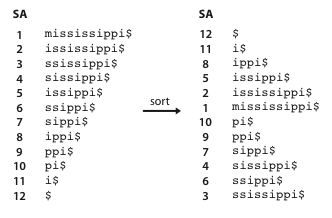# Burrows-Wheeler Transform The Burrows-Wheeler Transform (BWT) is a was developed by Burrows and Wheeler to reversibly permute a string in such a way that characters from repeated substrings would be clustered together. It was useful for compression schemes such as run-length encoding. It is not the point of this blog to explain how it works, but it is closely linked to Suffix Arrays:$BWT[i] = S[SA[i] – 1, BWT = \ (it wraps around) for the original string $S$, Suffix Array $SA$, and Burrows-Wheeler Transform string $BWT$. In other words, the $i^{th}$ symbol of the BWT is the symbol just before the $i^{th}$ suffix. See the image below: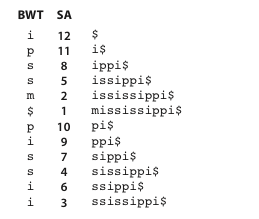In particular, $BWT = S[SA – 1] = S[12 – 1] = S = i$ (or the $11^{th}$ symbol from the original string 'mississippi').

Ferragina and Manzini (Ferragina et al. 2000) recommended that a BWT be paired with a Suffix Array, creating the so-called FM-Index, which enables backward search. The BWT also lets us reconstruct the original string $S$ (not covered in this blog), allowing us to discard the original document – indexes with this property are known as self indexes.

# Backward Search

This is where rank comes in. If it is hard to follow (it is certainly not easy to explain) then hang in there until the example, which should clear things up.

Since any pattern $P$ in $S$ (the original string) is a prefix of a suffix (our Suffix Array stores suffixes), and because the suffixes are lexicographically ordered, all occurrences of a search pattern $P$ lie in a contiguous portion of the Suffix Array. One way to hone in on our search term is to use successive binary searches. Storing the BWT lets us use a cooler way, though…

Backward search instead utilises the BWT in a series of paired rank queries (which can be answered with a Wavelet Tree, for example), improving the query performance considerably.
Backward search issues $p$ pairs of rank queries, where $p$ denotes the length of the pattern $P$. The paired rank queries are:

\begin{align} s^\prime &= C[P[i]] + rank(s-1, P[i]) + 1 \\ e^\prime &= C[P[i]] + rank(e, P[i]) \end{align}

Where $s$ denotes the start of the range and $e$ is the end of the range. Initially $s = 1$ and $e = N$. If at any stage $e \lt s$, then $P$ doesn’t exist in $S$.

As for $C$… $C$ is a lookup table containing the count of all symbols in our alphabet which sort lexicographically before $P[i]$. What does this mean? Well, $C$ would look like this: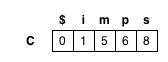Which means that there aren’t any characters in $S$ that sort before $\$$, one that sorts before i (the \$$), five that sort before$m$(the$\$$and the is) and so on. In the example I store it in a less compact way as the column F (which contains the first symbol for each suffix – essentially the same information, since each suffix is sorted), so it might be easier to follow (wishful thinking). Why is this called backwards search? Well, our index variable i actually starts at |P| (the last character of our search pattern), and decreases to 1. This maintains the invariant that SA[s..e] contains all the suffixes of which P[i..|P|] is a prefix, and hence all locations of P[i..|P|] in S. # Example Let’s practice this magic spell… Let our search pattern P be 'iss', and our string S be 'mississippi'. Starting with i = 3, c = P[i] = 's'. The working for each rank query is shown below each figure. I’m representing the current symbol as c to avoid confusion between 's' and s and s^\prime.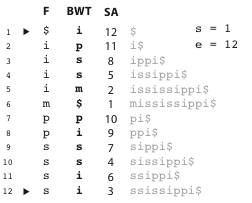Here (above) we are at the first stage of backwards search for 'iss' on 'mississippi' string – before any rank queries have been made. Note: we do not store the document anymore – the gray text – and we don’t store F, but instead store C – see section on Backward Search. Starting from s=1 and e=12 (as above) and c = P[i] = 's' where i = 3, we make our first two rank queries:$$
\begin{align}
s^\prime &= C[c] + rank(0, c) + 1 = 8 + 0 + 1 = 9 \\
e^\prime &= C[c] + rank(12, c) = 8 + 4 = 12
\end{align}
$$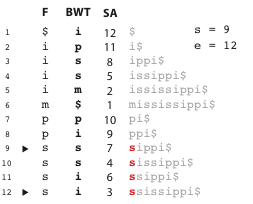After the above, we are now at the second stage of backwards search for 'iss' on 'mississippi' string. All the occurrences of 's' lie in SA[9..12]. From s = 9 and e = 11, and c = P[i] = 's' where i = 2, our next two rank queries are:$$
\begin{align}
s^{\prime\prime} &= C[c] + rank(8, c) + 1 = 8 + 2 + 1 = 11 \\
e^{\prime\prime} &= C[c] + rank(12, c) = 8 + 4 = 12
\end{align}
$$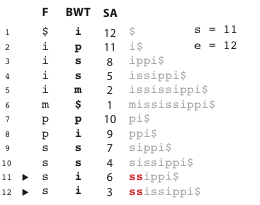We are now at the third stage of backwards search for 'iss' on 'mississippi' string. All the occurrences of 'ss' lie in SA[11..12]. From s = 11 and e = 12, and c = P[i] = 'i' where i = 1, our final two rank queries are:$$
\begin{align}
s^{\prime\prime\prime} &= C[c] + rank(10, c) + 1 = 1 + 2 + 1 = 4 \\
e^{\prime\prime\prime} &= C[c] + rank(12, c) = 1 + 4 = 5
\end{align}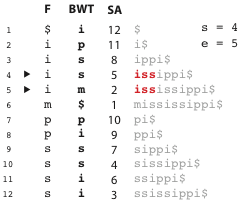This is the fourth and final stage of our backwards search for 'iss' in the string 'mississippi'. All the occurrences of 'iss' lie in $SA[4..5]$.

It impresses me every time…

# Play Time

No doubt you want to get your hands dirty. I have played around with libdivsufsort before, although I think you may have to implement backward search yourself (it’d be a good exercise), since it doesn’t appear to come with fast rank query providers. For rank structures for your BWT you might want to check out libcds. In fact there are heaps out there, but I haven’t used them yet.

Also, please comment here if you develop something cool with it :) and as always, if you have journeyed this far, consider following me on Twitter: @alexbowe.

# Bibliography

Ferragina, P. and Manzini, G. (2000). Opportunistic data structures with applications. Proceedings of the 41st Annual IEEE Symposium on Foundations of Computer Science, pages 390–398.

S. J. Puglisi, W. F. Smyth, and A. Turpin. A taxonomy of suffix array construction algorithms. ACM Computing Surveys, 39(2):1–31, 2007.
Jared Simpson’s paper from 2010 called *Efficient construction of an assembly string graph using the FM-index.

Simpson, J. T. and Durbin, R. (2010). Efficient construction of an assembly string graph using the FM-index. Bioinformatics, 26(12):i367–i373.